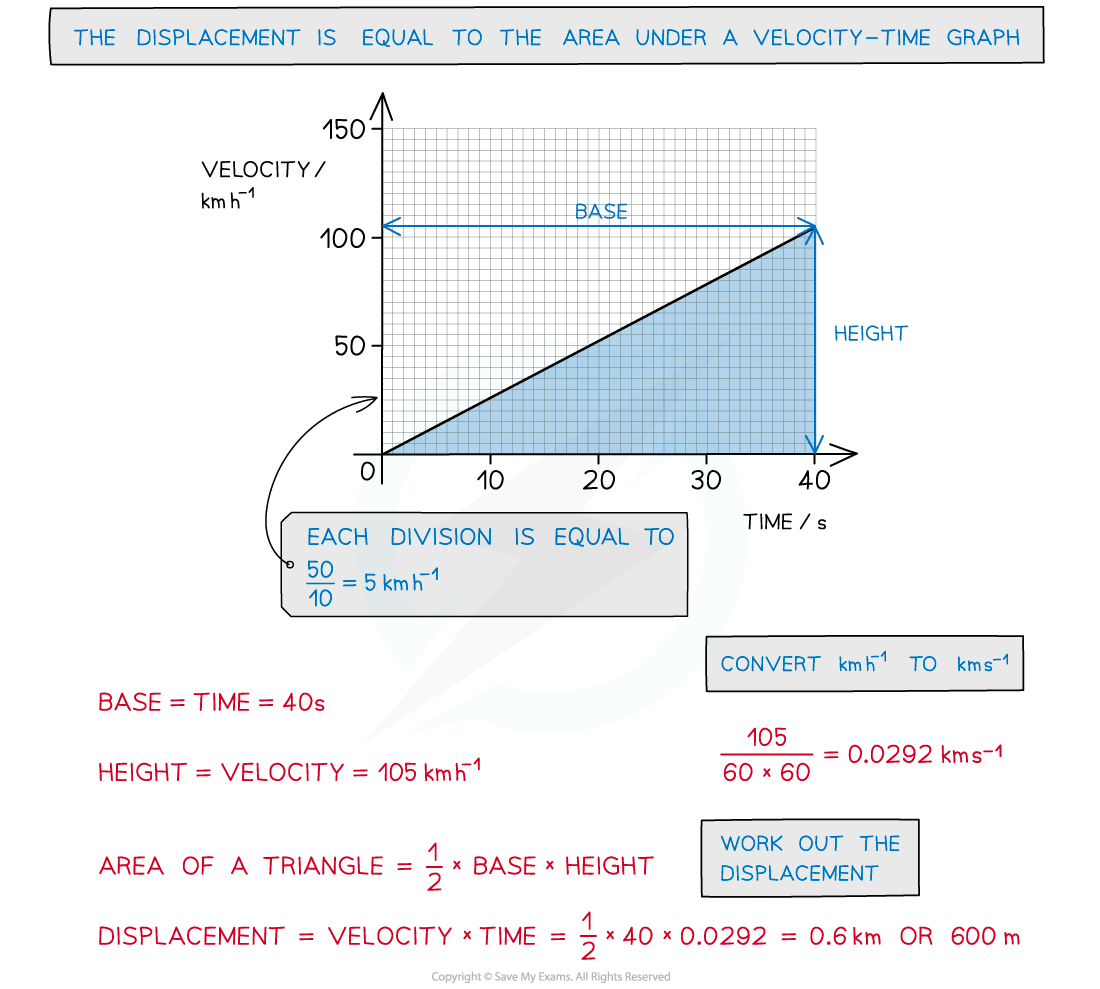# IB DP Physics: SL复习笔记2.1.4 Graphs Describing Motion

### Motion Graphs

• Three types of graphs that can represent motion are displacement-time graphs, velocity-time graphs, and acceleration-time graphs

#### Displacement-Time Graph

• On a displacement-time graph…
• Slope equals velocity
• The y-intercept equals the initial displacement
• A straight(diagonal) line represents a constant velocity
• A curved line represents an acceleration
• positive slope represents motion in the positive direction
• negative slope represents motion in the negative direction
• zero slope (horizontal line) represents a state of rest
• The area under the curve is meaningless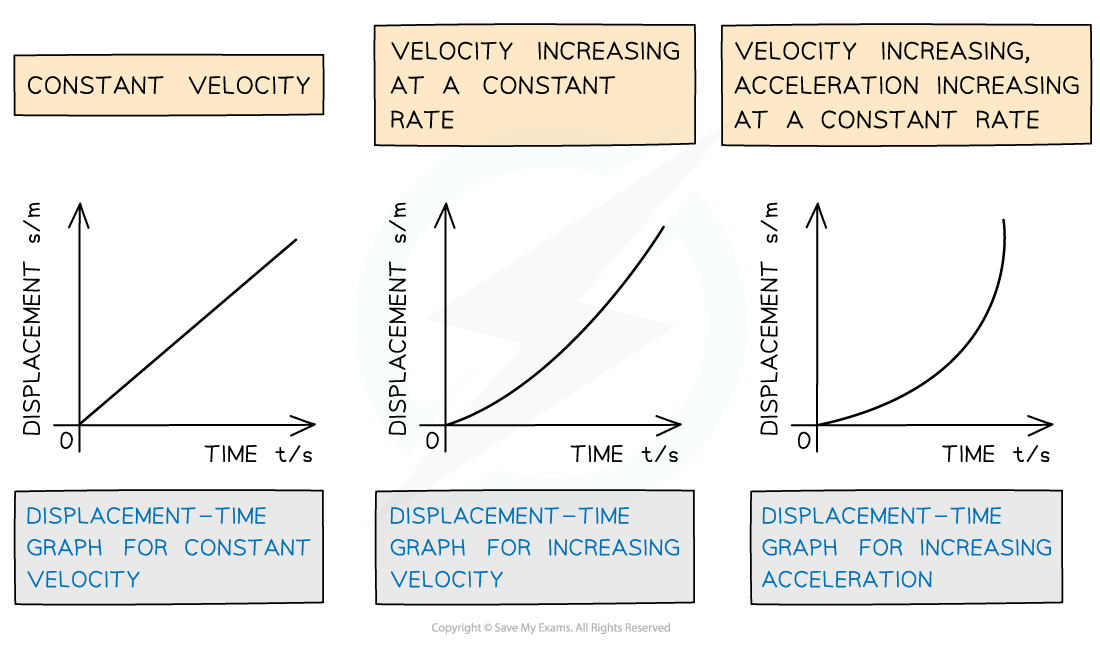Displacement-time graphs displacing difference velocities

#### Velocity-Time Graph

• On a velocity-time graph…
• Slope equals acceleration
• The y-intercept equals the initial velocity
• straight line represents uniform acceleration
• curved line represents non-uniform acceleration
• positive slope represents an increase in velocity in the positive direction
• negative slope represents an increase in velocity in the negative direction
• zero slope (horizontal line) represents motion with constant velocity
• The area under the curve equals the change in displacement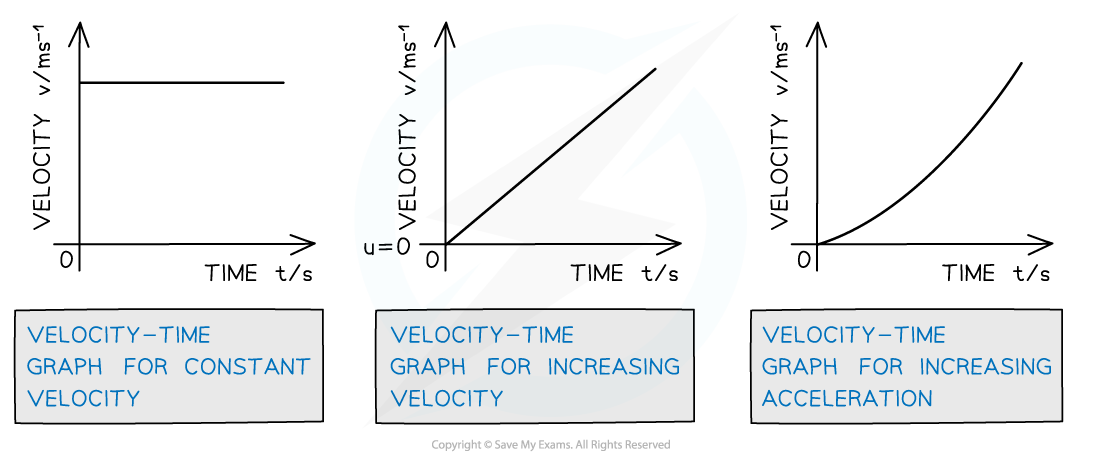Velocity-time graphs displacing different acceleration

#### Acceleration-Time Graph

• On an acceleration-time graph…
• Slope is meaningless
• The y-intercept equals the initial acceleration
• zero slope (horizontal line) represents an object undergoing constant acceleration
• The area under the curve equals the change in velocity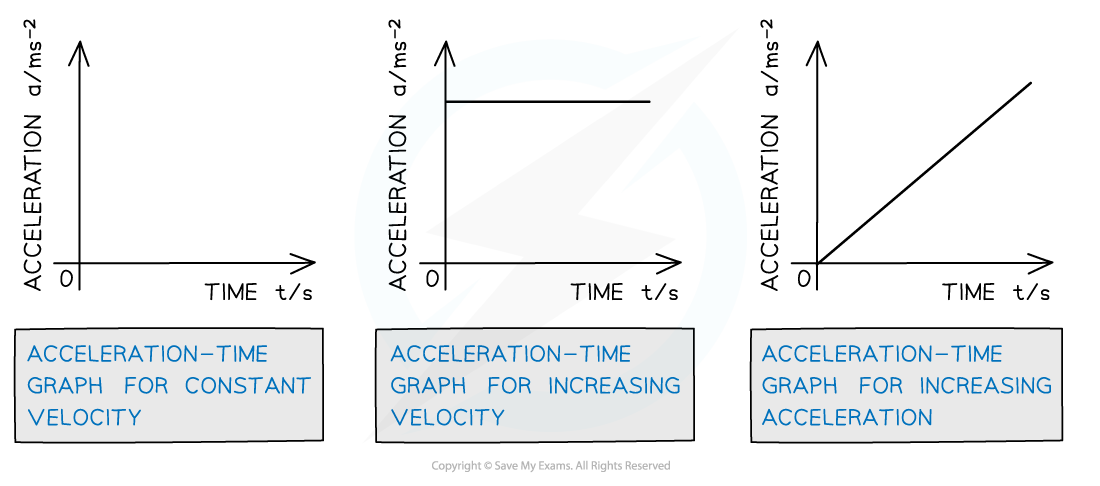How displacement, velocity and acceleration graphs relate to each other

#### Worked Example

Tora is training for a cycling tournament.

The velocity-time graph below shows her motion as she cycles along a flat, straight road.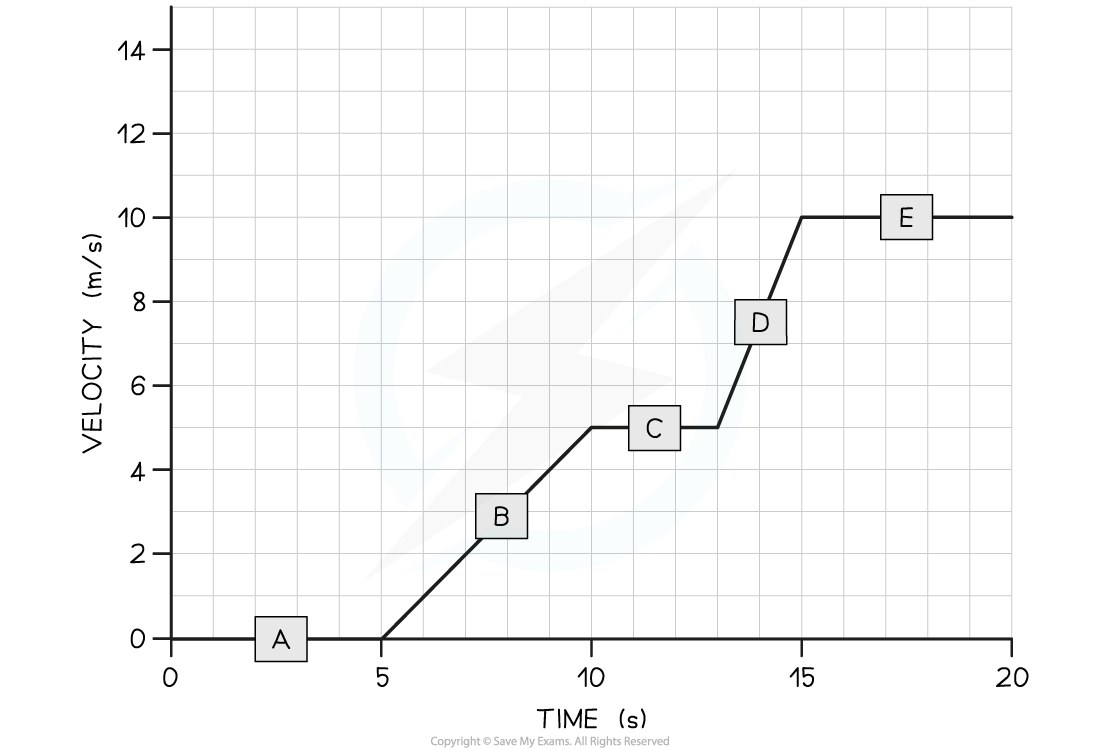(a) In which section (A, B, C, D, or E) of the velocity-time graph is Tora’s acceleration the largest?(b) Calculate Tora’s acceleration between 5 and 10 seconds.

Part (a)

Step 1: Recall that the slope of a velocity-time graph represents the magnitude of acceleration

• The slope of a velocity-time graph indicates the magnitude of accelerationTherefore, the only sections of the graph where Tora is accelerating is section B and section D
• Sections A, C, and E are flat – in other words, Tora is moving at a constant velocity (i.e. not accelerating)

Step 2: Identify the section with the steepest slope

• Section D of the graph has the steepest slope
• Hence, the largest acceleration is shown in section D

Part (b)

Step 1: Recall that the gradient of a velocity-time graph gives the acceleration

• Calculating the gradient of a slope on a velocity-time graph gives the acceleration for that time period

Step 2: Draw a large gradient triangle at the appropriate section of the graph

• A gradient triangle is drawn for the time period between 5 and 10 seconds below: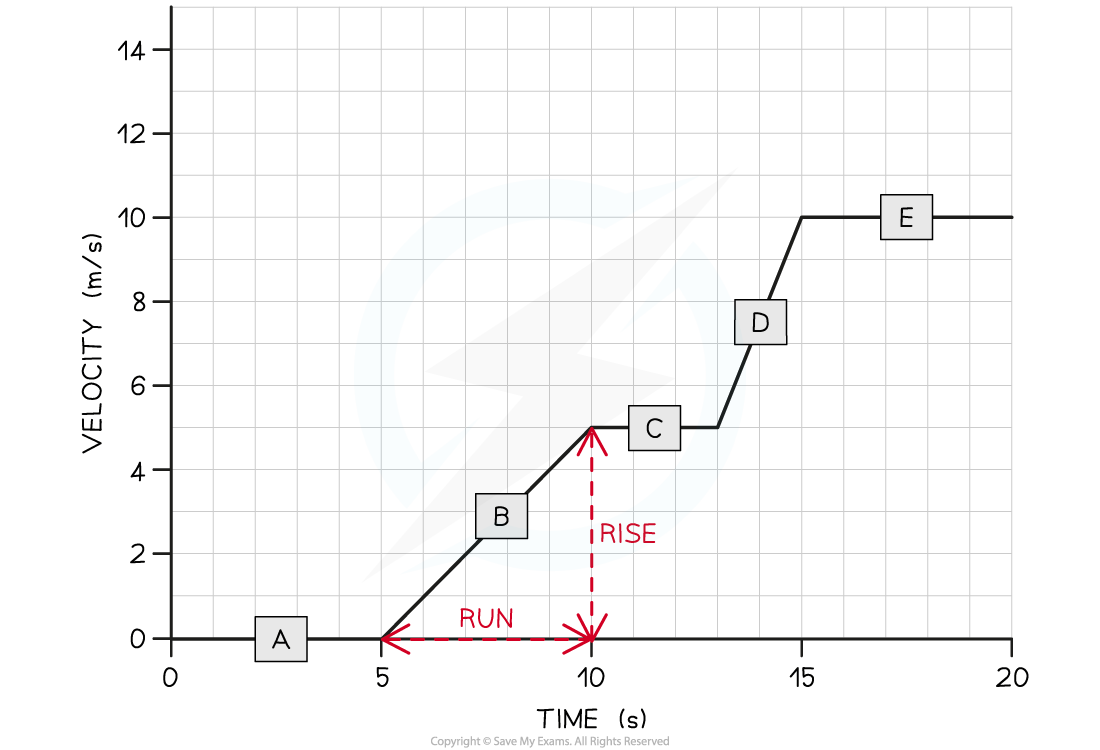Step 3: Calculate the size of the gradient and state this as the acceleration

• The acceleration is given by the gradient, which can be calculated using:

acceleration = gradient = 5 ÷ 5 = 1 m/s2

• Therefore, Tora accelerated at 1 m/s2 between 5 and 10 seconds

#### Motion of a Bouncing Ball

• For a bouncing ball, the acceleration due to gravity is always in the same direction (in a uniform gravitational field such as the Earth's surface)
• This is assuming there are no other forces on the ball, such as air resistance
• Since the ball changes its direction when it reaches its highest and lowest point, the direction of the velocity will change at these points
• The vector nature of velocity means the ball will sometimes have a:
• Positive velocity if it is traveling in the positive direction
• Negative velocity if it is traveling in the negative direction
• An example could be a ball bouncing from the ground back upwards and back down again
• The positive direction is taken as upwards
• This will be either stated in the question or can be chosen, as long as the direction is consistent throughout
• Ignoring the effect of air resistance, the ball will reach the same height every time before bouncing from the ground again
• When the ball is traveling upwards, it has a positive velocity which slowly decreases (decelerates) until it reaches its highest point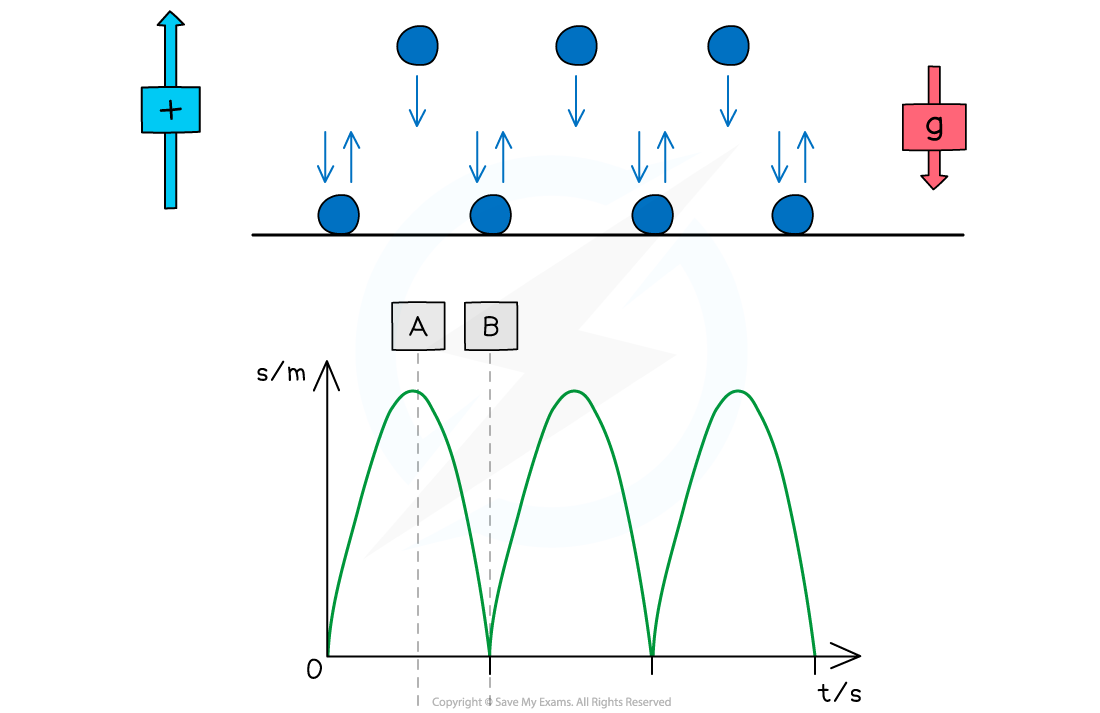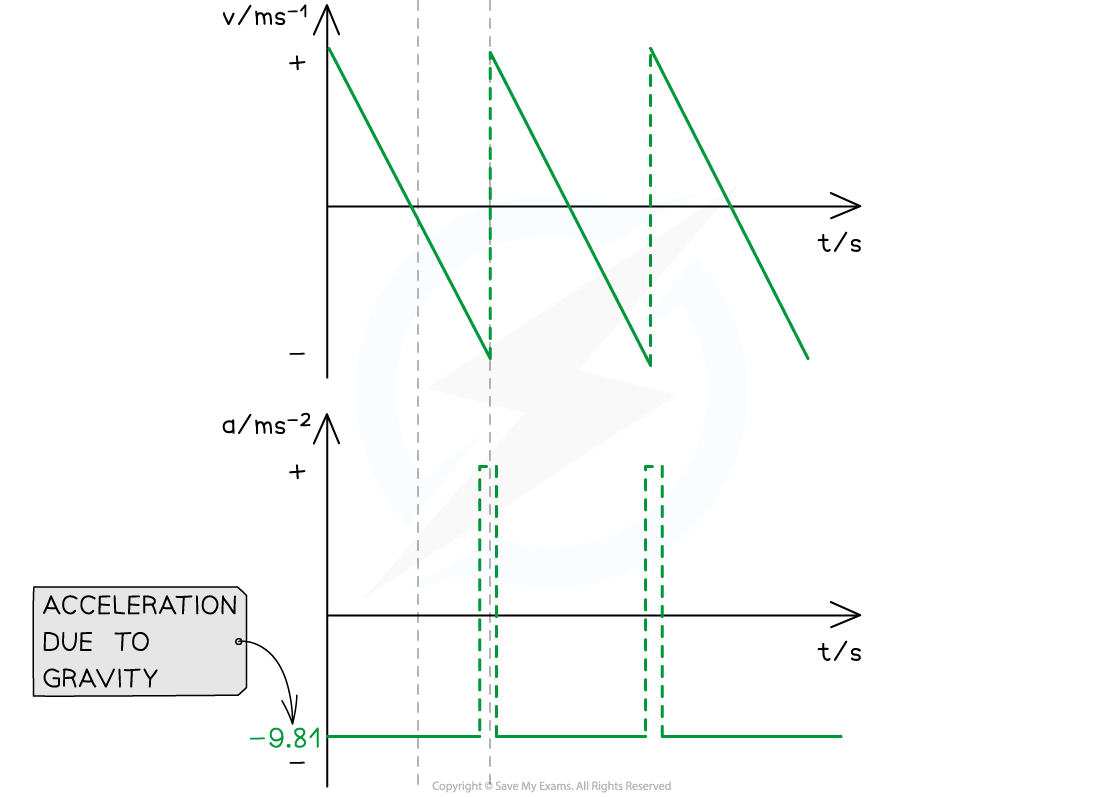• At point (the highest point):
• The ball is at its maximum displacement
• The ball momentarily has zero velocity
• The velocity changes from positive to negative as the ball changes direction
• The accelerationg, is still constant and directed vertically downwards
• At point (the lowest point):
• The ball is at its minimum displacement (on the ground)
• Its velocity changes instantaneously from negative to positive, but its speed (magnitude) remains the same
• The change in direction causes a momentary acceleration (since acceleration = change in velocity / time)

#### Worked Example

The velocity-time graph of a vehicle travelling with uniform acceleration is shown in the diagram below.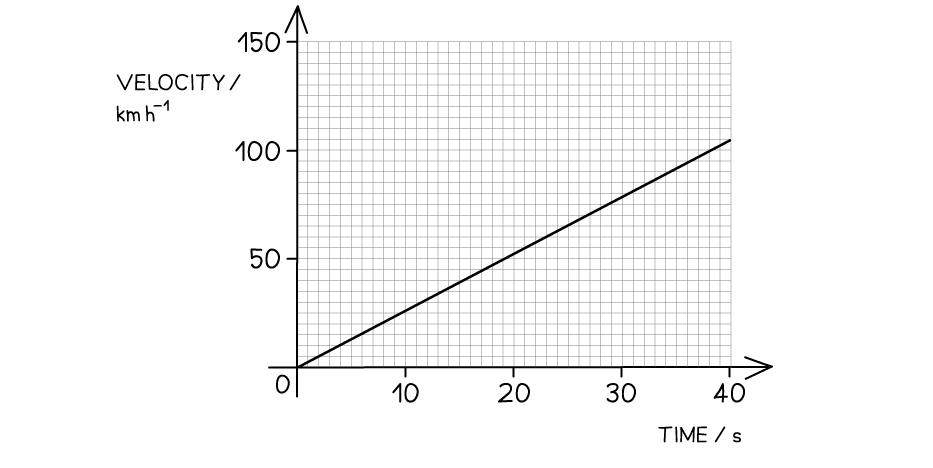Calculate the displacement of the vehicle at 40 s.